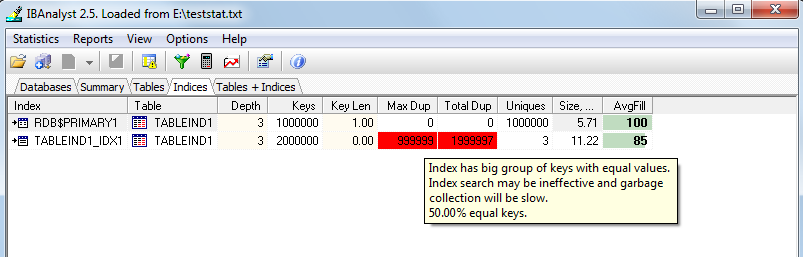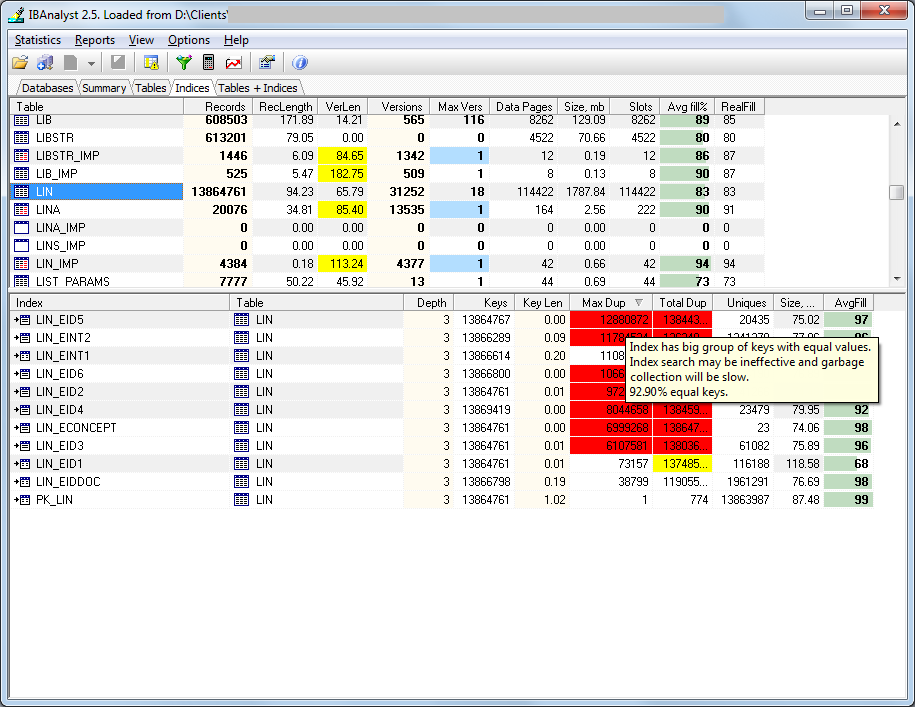# Library

## Negative impact of indices to INSERT, UPDATE and DELETE performance in Firebird SQL

In general, indices are necessary for any serious database, since they are critical to speed up queries with WHERE clause (SELECT, UPDATE. MERGE, DELETE, etc). However, every index has the cost, and the cost is obvious when we compare the speed INSERT/UPDATE/DELETE operations on indexed and non-indexed fields.

In this article, we will demonstrate the impact of the index with a few unique keys to the operations' speed.

## Test database

Let's create the simple Firebird database:
```CREATE DATABASE “E:\TESTFIREBIRDINDEX.FDB” USER “SYSDBA” PASSWORD “masterkey”;
CREATE TABLE TABLEIND1 (
I1            INTEGER NOT NULL PRIMARY KEY,
NAME          VARCHAR(250),
MANORWOMAN  SMALLINT
);
CREATE GENERATOR G1;
SET TERM ^ ;

create or alter procedure INS1MLN
returns (
INSERTED_CNT integer)
as
BEGIN
inserted_cnt = 0;
WHILE (inserted_cnt <1000000) DO
BEGIN
Insert into tableind1(i1, name, manorwoman) values(gen_id(g1,1), 'TEST name', (:inserted_cnt - (:inserted_cnt/2)*2));
inserted_cnt=inserted_cnt+1;
END
suspend;
END^
SET TERM ; ^
GRANT INSERT ON TABLEIND1 TO PROCEDURE INS1MLN;
GRANT EXECUTE ON PROCEDURE INS1MLN TO SYSDBA;
COMMIT;
```

To demonstrate the problem with bad indices let's perform the following operations:

• First, we insert 1 million of records into the table
• Then we update these records
• After that, we delete all records
• And, finally, run SELECT count(*) from the table
The commands below perform the described operations:
```set stat on;  /*enable display of statistics in isql*/
select * from ins1mln;
update tableind1 SET MANORWOMAN  = 3;
delete from tableind1;
select count(*) from tableind1;
```

Let's run it and keep the results for further analysis.

After that let's create another table with the same structure - and add there the index for MANORWOMAN column
```CREATE INDEX TABLEIND1_IDX1 ON TABLEIND1 (MANORWOMAN);
```

As you can see above in the script, we insert to this column only 0 or 1 integer values. Such index is useless for select queries, and in theory, all database developers should avoid such bad indices (except very special case with an unbalanced distribution of values in the table), but in practice, there are many indices with 2 unique values or even with 1 of them.

Then let's repeat the script with this index and compare results – see the following table:

 Without index for MANORWOMAN With index for MANORWOMAN SQL> set stat on; /*show statistics*/ SQL> select * from ins1mln;   INSERTED_CNT ============      1000000   Current memory = 10487216 Delta memory = 80560 Max memory = 12569996 Elapsed time= 13.33 sec Buffers = 2048 Reads = 0 Writes 18756 Fetches = 7833503 SQL> update tableind1 SET MANORWOMAN = 3; Current memory = 76551788 Delta memory = 66064572 Max memory = 111442520 Elapsed time= 15.04 sec Buffers = 2048 Reads = 16166 Writes 15852 Fetches = 6032307 SQL> delete from tableind1; Current memory = 76550240 Delta memory = -1548 Max memory = 111442520 Elapsed time= 3.27 sec Buffers = 2048 Reads = 16147 Writes 16006 Fetches = 5032277 SQL> select count(*) from tableind1;          COUNT ============            0   Current memory = 76552064 Delta memory = 1824 Max memory = 111442520 Elapsed time= 1.35 sec Buffers = 2048 Reads = 16021 Writes 1791 Fetches = 2032278 SQL> set stat on; /*show statistics*/ SQL> select * from ins1mln;   INSERTED_CNT ============      1000000   Current memory = 10484140 Delta memory = 75524 Max memory = 12569996 Elapsed time= 23.94 sec Buffers = 2048 Reads = 1 Writes 23942 Fetches = 11459599 SQL> update tableind1 SET MANORWOMAN = 3; Current memory = 76548712 Delta memory = 66064572 Max memory = 111439444 Elapsed time= 29.30 sec Buffers = 2048 Reads = 16167 Writes 19492 Fetches = 10035948 SQL> delete from tableind1; Current memory = 76547164 Delta memory = -1548 Max memory = 111439444 Elapsed time= 3.41 sec Buffers = 2048 Reads = 16147 Writes 15967 Fetches = 5032277 SQL> select count(*) from tableind1;          COUNT ============            0   Current memory = 76548988 Delta memory = 1824 Max memory = 111439444 Elapsed time= 0.69 sec Buffers = 2048 Reads = 16021 Writes 1901 Fetches = 2032278

So, bad index decreases performance by approximately 2 times while inserting or updating. Also, we can see that non-optimal index greatly increases the number of writes and record fetches.

Let’s get statistics for this sample database (with the bad index for MANORWOMAN) and try to find some details. To gather statistics, we run the following command:

```gstat -r e:\testfirebirdindex.fdb > e:\teststat.txt
```
TABLEIND1 table and indices statistics section look intriguing, but what useful information it gives to us?
```TABLEIND1 (128)
Primary pointer page: 166, Index root page: 167
Average record length: 0.00, total records: 1000000
Average version length: 27.00, total versions: 1000000, max versions: 1
Data pages: 16130, data page slots: 16130, average fill: 93%
Fill distribution:
0 - 19% = 1
20 - 39% = 0
40 - 59% = 0
60 - 79% = 0
80 - 99% = 16129

Index RDB\$PRIMARY1 (0)
Depth: 3, leaf buckets: 1463, nodes: 1000000
Average data length: 1.00, total dup: 0, max dup: 0
Fill distribution:
0 - 19% = 0
20 - 39% = 0
40 - 59% = 0
60 - 79% = 1
80 - 99% = 1462

Index TABLEIND1_IDX1 (1)
Depth: 3, leaf buckets: 2873, nodes: 2000000
Average data length: 0.00, total dup: 1999997, max dup: 999999
Fill distribution:
0 - 19% = 0
20 - 39% = 1
40 - 59% = 1056
60 - 79% = 0
80 - 99% = 1816

```

To understand the meaning of shown numbers and percentage values, we can use HQbird Database Analyst, which offers visual interpretation of database statistics:By clicking Reports/View recommendations we can find the appropriate explanation for this index: